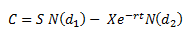Black-Scholes Option Model

Posted in Finance, Accounting and Economics Terms, Total Reads: 2299

Definition: Black-Scholes Option Model

Black-scholes option pricing model is another model which is widely used to compute the price of European call options. It assumes that the price of the underlying follows a geometric Brownian motion with constant drift and velocity whereas in case of stocks it assumes constant price variation of stock, time value of money etc.

It uses the concept of exercise price along with time value of money to compute the price of the option.

The pricing model has a complex formula. It is computed as:Where

C = Price of the call option

S = current price of the underlying

X = exercise price

R = risk free rate

T = time until expiration of option

N() = area under the normal curve

Browse the definition and meaning of more terms similar to Black-Scholes Option Model. The Management Dictionary covers over 7000 business concepts from 6 categories.

Search & Explore : Management Dictionary

Similar Definitions from same Category: